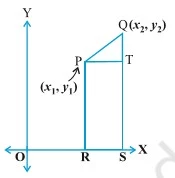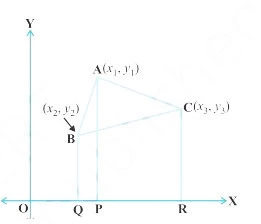Click Question to Get Free Answers >

# Coordinate Geometry NCERT Solutions : Class 10 Maths

## NCERT Solutions for Class 10 Maths : Coordinate GeometryFilter
Filters :
Classes
•
•
•
•
•
•
•
Chapters
•
•
•
•
•
•
•
•
•
•
• 4 More
Exercises
•
•
•
•
•

### NCERT Class 10 | COORDINATE GEOMETRY | Exercise 04 | Question No. 08

ABCD is a rectangle formed by the points `A(1,"\ "1),"\ "B(1,"\ "4),"\ "C(5,"\ "4)"\ "a n d"\ "D(5,"\ "1)` . P, Q, R and S are the midpoints of AB, BC, CD and DA respectively. Is the quadrilateral PQRS a square? A rectangle? or a rhombus? Justify yoDoubtnut one of the best online education platform provides free NCERT Solutions of Maths for Class 10 Coordinate Geometry which are solved by our maths experts as per the NCERT (CBSE) guidelines. We provide the solutions as video solutions in which concepts are also explained along with answer which will help students while learning , preparing for exams and in doing homework. These Solutions will help to revise complete syllabus and score more marks in examinations.

Get here free, accurate and comprehensive NCERT Solutions for Class 10 Maths Coordinate Geometry which have been reviewed by our maths counsellors as per the latest edition following up the CBSE guidelines. We provide video solutions in which solutions to all the questions of NCERT Class 10 Maths textbook are explained in step by step and detailed way.

## The Topics of the Chapter Coordinate Geometry are :

SECTION FORMULA

DISTANCE B/W TWO POINTS

AREA OF TRIANGLE

INTRODUCTION

SOME APPLICATIONS OF SECTION FORMULA

It contains these exercises along with solved examples. All the exercises are solved in the video. Select the exercise to view the solutions exercisewise of the Chapter Coordinate Geometry:

## NCERT Solutions Class 10 Maths Chapter Coordinate Geometry Exercises:

We have covered all the exercises and also Solved examples in the videos. Along with the practise exercise Students should also practise solved examples to clear the concepts of the Coordinate Geometry. If incase you have any doubt you can watch the solutions for the given questions.Watch the solutions for the given questions in which it is also explained in the video steps to solve the questions along with answers.

In the NCERT Class 10 chapter Coordinate Geometry, we will focus on different topics including distance formula, section formula, area of a Triangle. It becomes quite easy for the students to get all the right information on how to find the right solutions of the different problems. Extra effort has been made by the team of Doubtnut in getting the accurate idea as to how to clear all the queries in the right manner.

It has been noticed that NCERT textbooks helps to provide the right information on the basic topics. However, students find it quite problematic when it comes to attempting the different tricky questions. The questions either appear to be quite difficult or the students get confused due to which scoring good marks prove to be a dream for them. Therefore, it is seen that students try to look forward to the various guides that are available in the market. This helps them to clear their queries to a certain extent.

Taking into considerations of all the problems faced by the students, a team of experts of Doubtnut has come up with a unique idea to solve it. They have made the chapters quite interesting by providing the best online study material. The best part is that students can access these video tutorials online no matter where they are and that too free of cost as well. Thus, this proves to be quite interesting for them where they can get the perfect idea as to how it would be possible to attempt the different questions in the easiest approach. It is also possible to access NCERT Solutions for class 10 maths with the help of their experts.

## Topics and Subtopics included in NCERT Solutions for Class 10 Maths Chapter 7 Coordinate Geometry

### Introduction

You are already familiar that it requires a pair of coordinate axes in order to locate position of a point on a plane. ‘x-coordinate’ or ‘abscissa’ is the distance of a point from the x-axis. While, ‘y-coordinate’ or ‘ordinate’ is the distance of a point from the y-axis. Here, in this chapter, it would be possible to find the distance between the two points when the coordinates are given.

### Distance Formula

It is possible to find the distance between two points. Let’s have a look at an example.Let us now find the distance between any two points P (x1, y1) and Q(x2, y2).

Draw PR and QS perpendicular to the x-axis. A perpendicular from the point P on QS is drawn to meet it at the point T.

Then, OR = x1, OS = x2. So, RS = x2 – x1 = PT.

Also, SQ = y2, ST = PR = y1.

So, QT = y2 – y1.

Now, applying the Pythagoras theorem in âˆ† PTQ, we get

PQ2 = PT2 + QT2

= (x2 – x1)2 + (y2 – y1)2

Therefore, PQ = square root of (x2 – x1)2 + (y2 – y1)2

Note that since distance is always non-negative, we take only the positive square root. So, the distance between the points P(x1, y1) and Q(x2, y2) is

PQ = square root of (x2 – x1)2 + (y2 – y1)2 which is known as the distance formula.

### Section Formula

Finding the right solution for section formula is possible by making use of the coordinates. In order to get the right and clear idea, let’s have a look at an example.

Find the coordinates of the point which divides the line segment joining the points (4, – 3) and (8, 5) in the ratio 3:1 internally.

Solution: Let P(x, y) be the required point. Using the section formula, we get

x = 3(8) + 1(4) = 7, y = 3(5) + 1(-3) =3

3+1                         3 +1

Therefore, (7, 3) is the required point.

### Area of a Triangle

You are familiar on how to calculate the area of a triangle when its base and corresponding height are given. The formula that you have used was:

Area of a triangle = 1 x base x altitude

2

You also learnt Heron’s formula in order to know the area of a triangle. But do you think that there is any other easier method?Let ABC be any triangle whose vertices are A (x1, y1), B (x2, y2) and C (x3, y3). Draw AP, BQ and CR perpendiculars from A, B and C, respectively, to the x-axis. Clearly ABQP, APRC and BQRC are all trapezia.

Now, from the above figure it is clear that

area of âˆ† ABC = area of trapezium ABQP + area of trapezium APRC – area of trapezium BQRC.

It is also known that the

Area of a trapezium = ½ (sum of parallel sides) (distance between them)

Therefore,

Area of âˆ† ABC = ½ (BQ+AP) QP+ ½ (AP+CR) PR – ½ (BQ + CR) QR

= ½ (y2 +y1) (x1-x2) + ½ (y1 + y3) (x3-x1) – ½ (y2 + y3) (x3-x2)

= ½ [x1(y2 - y3) + x2(y3 – y1) +x3(y1 - y2)]

Thus, the area of âˆ† ABC is the numerical value of the expression

½ [x1(y2 - y3) + x2(y3 – y1) +x3(y1 - y2)]

## How is Doubtnut helpful for Class 10 students?

Doubtnut has truly made it possible to impart the best and easiest solution for the students. The NCERT Class 10 Maths Solutions Chapter 7 has really become quite easier for them to get rid of all their queries.  There are also lots of other advantages that can be seen when students opt for the useful video tutorials developed by the experts of Doubtnut.

• Easy to learn different concepts:   It becomes quite easier for the class 10 CBSE boards students to learn different topics with ease. This is possible because the experts have made it quite easy in explaining the concepts. This does not lead to feeling any sort of problem in working on the different questions. So, students are able to get the perfect idea on the different question patterns which make them much easier for them to solve even the toughest questions. So, it proves to be quite helpful for the students in getting all important information as to how to come up with the right solution.
• Get lots of exercises and examples: The team of expert tutors has really put a lot of effort in providing lots of different useful exercises and examples. This helps students to get the best practice so that it helps in making them expert in the subject. It also helps to boost the confidence of the students where they can find it much easier to complete a certain task in the best way.
• Expect ultimate support: The team of Doubtnut helps to provide the best support for which the students are able to stay away from any sort of confusions at all. Therefore, students who wish to score good marks in Maths should definitely try to look forward to the best and useful video tutorials by Doubtnut.